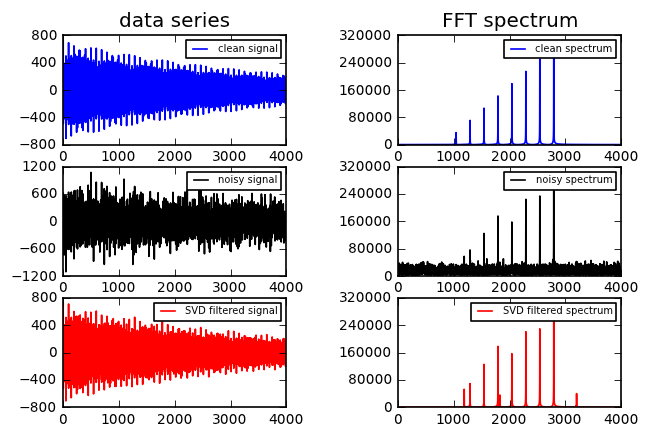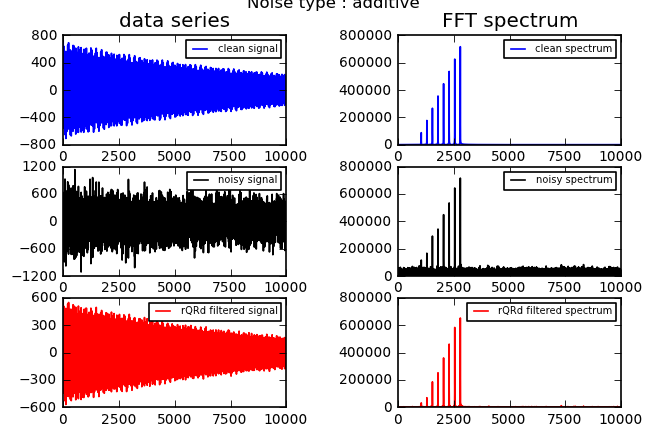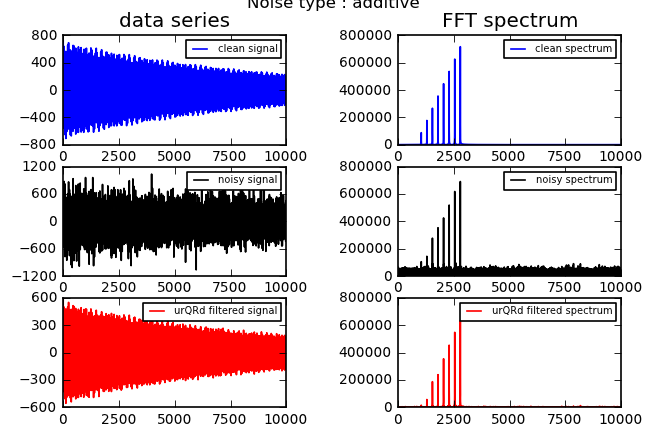To run the several examples, simply click in the code cell, and type shift-ENTER.

# Examples¶

This file implements several examples of the de-noising algorithms Cadzow rQRd and urQRd.

First example runs a test of the Cadzow de-noising method, based on the SVD decomposition of a matrix derived from the data-set (see theory).

From the experimental data $X_i$, a Hankel matrix $H$ is built:

$$(H_{ij}) = (X_{i+j-1})$$

$H$ is rank-limited to $P$ in the absence of noise. In noisy data-sets, this matrix becomes full-rank because of the partial decorrelation of the data-points induced by the noise.

Cadzow (Cadzow JA (1988) IEEE Trans. ASSP 36 49–62.) proposed to perform the Singular Value Decomposition ( _see SVD on Wikipedia_ ) of the matrix $H$, and compute a matrix $\tilde{H}$ by truncating to the $K$ largest singular values $\sigma_k$.

$$H = U \Lambda V$$

where $\Lambda$ is a diagonal matrix containing the singular values of $H$.

$$\tilde{H} = U \Lambda_k V$$

where $\Lambda_k$ contains only the $k$ largest singular values.

$\tilde{H}$ is not strictly Hankel-structured anymore, but a de-noised signal $\tilde{X}$ can be reconstructed by taking the average of all its antidiagonals.

$$\tilde{X_{l}} = < \tilde{H_{ij}} >_{i+j=l+1}$$

## Running the example¶

Figure presents the data-set on the left, and its Fourier transform on the right.

The data-set is composed of 8 equidistant frequencies of different intensities. Gaussian noise is added to the data.

call is and default values are :

def test_Cadzow(
lendata = 4000,  # length of the simulated data
rank = 10,       # rank at which the analysis is performed
orda = 1600,     # order of the analysis, the Hankel matrix is (lendata-orda) X orda
noise = 200.0,   # level of noise in the simulated data
iteration=1,     # the algorithm can be iterated several times for better (slower!) results
noisetype = "additive")   # possible noisetype are 'additive' 'multiplicative' 'sampling' 'missing points'



you can test_Cadzow() with modifying any parameter

In :
%pylab inline
params = {'savefig.dpi':120,'legend.fontsize':6,'xtick.labelsize':'small','ytick.labelsize':'small','figure.subplot.wspace':0.5}
rcParams.update(params)

Welcome to pylab, a matplotlib-based Python environment [backend: module://IPython.zmq.pylab.backend_inline].
=== Running Cadzow algo === lendata: 4000  orda: 1600  rank: 10
=== Result ===
Denoised SNR: 14.43 dB  - processing gain : 18.15 dB
processing time for Cadzow : 5.65 sec## B) rQRd¶

rQRd is based on a random sampling of $H$ by a random matrix $\Omega$ : $$\underset{(M \times K)}{Y} = \underset{(M \times N)}{H} \underset{(N \times K)}{\Omega}$$ The matrix $Y$ is much smaller than $H$, it can rapidly by factorized by QR ( _see QR on Wikipedia_ ):

$$Y = QR$$

from which a rank-truncated $\tilde{H}$ is built by projection of the subspace defined by $Q$ : $$\tilde{H} = QQ^*H$$

$\tilde{X}$ is then computed as before.

This second example runs the same test using rQRd. Note that test test here is run on 10.000 points rather than 4.000

default values are :

def test_rQRd(  lendata = 10000,
rank=100,
orda=4000,
noise = 200.0,
iteration = 1,
noisetype = "additive")
In :
from Algorithms import rQRd
rQRd.test_rQRd()

Initial Noisy Data SNR: -3.89 dB - noise type : additive
=== Running rQR algo === lendata: 10000  orda: 4000  rank: 100
=== Result ===
Denoised SNR: 13.45 dB  - processing gain : 17.34 dB
processing time for rQRd : 2.27 sec## C) urQRd¶

urQRd is based on the same grounds as rQRd but contrarily to rQRd, it makes use of fast matrix-matrix products.

Those fast products are made possible thanks to classical FFT techniques using convolution products. For urQRd, the product $H\Omega$ has a cost of $KL$log$(L)$.

The matrix $Y$ is much smaller than $H$, it can rapidly be factorized by QR ( _see QR on Wikipedia_ ) : $Y = QR$

A second product is necessary for urQRd : $Q^*H$. In this case the product has a cost close to $KL$log$(L)$ when using the fast structured matrix vector product.

$\tilde{X}$ the denoised signal is then computed from $Q$ and $Q^*H$ using FFT again and indices inversion.

Let $U = Q^*H$, each $\tilde{X_i}$ is obtained from the $i^{th}$ (${n_i}$ elements long) antidiagonal by :

$$\tilde{X_i} = \frac{1}{n_i}\sum_{j=max(i-M+1,1)}^{min(i,N)} H_{i-j+1,j}\approx \frac{1}{n_i} \sum_{k=1}^{K} \sum_{j=max(i-M+1,1)}^{min(i,N)} Q_{i-j+1,k}U_{k,j}$$$$\;\;\;\;\;\;\;\;\; = \frac{1}{n_i} \sum_{k=1}^{K} \sum_{j=max(i-M+1,1)}^{min(i,N)} Q_{i,j}^{(k)}U_{j}^{(k)} = \frac{1}{n_i} \sum_{k=1}^{K} (Q^{(k)}.U^{(k)})_i$$

$Q^{(k)}$ is the $L \times N$ Toeplitz matrix formed with the vector $[0,....0,X_{0},X_{1},..X_{L},0,....,0]^T$ with $(N-1)$ zeros at each extremity of the vector.

$U^{(k)}$ is the matrix $U_{i,j}$ This last operation has a cost of $K(L+N)$log$(L+N)$.

urQRd scales globally in $KN$log$(N)$.

All those fast calculations are based on the FFT fast matrix-vector product.

Fast Hankel Matrix vector product

Let $H$ be a Hankel matrix of dimensions $M \times N$, $g$ its generator vector of size $M+N-1$ and $v$ a vector of size $M$.

$w$ is a vector of size $M+N-1$ defined from $v$ as :

$w_i = v_{i}$ for $1 \leq i \leq N$ and $w_i = 0$ for $N+1 \leq i \leq M+N-1$

let $P$ be the swapping matrix that swaps vector element $i$ with element $M+N-i$.

The product Hankel matrix H with vector v, $Hv$ is made faster by performing the calculation:

$Hv = FFT^{-1}(FFT(g).FFT(P.w))$

default values are :

def test_urQRd(
lendata = 10000,
rank = 100,
orda = 4000,
noise = 200.0,
iteration = 1,
noisetype = "additive")
In :
from Algorithms import urQRd
urQRd.test_urQRd()

Initial Noisy Data SNR: -4.00 dB - noise type : additive
=== Running urQR algo === lendata: 10000  orda: 4000  rank: 100
=== Result ===
Denoised SNR: 13.22 dB  - processing gain : 17.22 dB
processing time for urQRd : 0.42 sec## Remark¶

If you want to have a look the code used here, change the definition of prgm and execute the cell.

In :
prgm = "rQRd.py"  # "rQRd.py" "urQRd.py" "Cadzow.py"

# depending on your version of IPython, one of the two following line might be used, choose your own
import IPython.core.display as disp # see remark above
#import IPython.display as disp # see remark above
#
from pygments import highlight
from pygments.lexers import PythonLexer
from pygments.formatters import HtmlFormatter
formatter = HtmlFormatter(noclasses=True)
css = formatter.get_style_defs('.highlight')
code = ''.join(list(open("Algorithms/"+prgm)))
disp.HTML(highlight(code, PythonLexer(), formatter))

In [ ]: